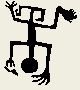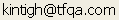Tools for Quantitative Archaeology    Email:POISSON: Compute Poisson and Negative Binomial Probabilities

POISSON prints poisson and negative binomial probabilities given expected counts.The program will optionally calculate expected counts for a sample from a population density and a sample unit size (in the units of the density measure). In the latter case, the expected count is simply the population density*sample unit size. For the negative binomial distribution, a parameter (r) is requested. The parameter r must be a positive integer. The lower the r, the more clustered the distribution. The program outputs only those probabilities > .0005. The program cannot calculate probabilities for counts greater then about 400 (in which case you probably don't want to be doing this anyway) because of the very large numbers involved.

SAMPLE PROGRAM RUN

```Output File Name {CON} ?
Poisson/Negative Binomial Probabilities

Enter [E]xpected Counts or [D]ensity & Sample Interval {E} ? E
[P]oisson (random) or [N]eg. Binomial (clustered) {P} ? P
Expected Count Per Sample Interval {   1.0000} ?
Observed Sample Count {0} ? 3
Poisson Probability Calculation
Expected Count          1.0000
Observed Count          3

Count | Prob(Cnt)  Prob(<=Cnt)  Prob(>=Cnt)
0 |   0.368       0.368        1.000
1 |   0.368       0.736        0.632
2 |   0.184       0.920        0.264
>   3 |   0.061       0.981        0.080
4 |   0.015       0.996        0.019
5 |   0.003       0.999        0.004
6 |   0.001       1.000        0.001```

This analysis indicates that under a Poisson model, if the mean (expected) count per sample interval is 1, then the probability of finding exactly three in any interval is .06, and the cumulative probability of finding three or fewer is .98.

```Repeat {Y} ?
[P]oisson (random) or [N]eg. Binomial (clustered) {P} ? N
Negative Binomial Parameter {1} ?
Expected Count Per Sample Interval {   1.0000} ?
Observed Sample Count {3} ?
Negative Binomial (1) Probability Calculation
Expected Count          1.0000
Observed Count          3

Count | Prob(Cnt)  Prob(<=Cnt)  Prob(>=Cnt)
0 |   0.500       0.500        1.000
1 |   0.250       0.750        0.500
2 |   0.125       0.875        0.250
>   3 |   0.063       0.938        0.125
4 |   0.031       0.969        0.063
5 |   0.016       0.984        0.031
6 |   0.008       0.992        0.016
7 |   0.004       0.996        0.008
8 |   0.002       0.998        0.004
9 |   0.001       0.999        0.002

Repeat {Y} ? N
Program End```

Similarly, this analysis indicated that if the counts are clustered to the degree specified by a negative binomial distribution with r=1, the probability of finding exactly three counts in a sample interval with a mean of 1 is also about .06. However, note that the distribution is more spread out than that of the Poisson distribution. Counts both smaller (e.g. 0) and larger (e.g. 5) than the mean are more likely under a clustered model (this is essentially true by definition).

 Home Top Overview Ordering Documentation

Page Last Updated - 02-Jun-2007